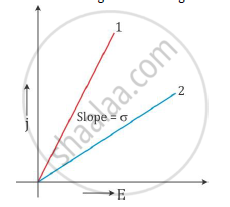# Plot a Graph Showing the Variation of Current Density (J) Versus the Electric Field (E) - Physics

plot a graph showing the variation of current density (j) versus the electric field (E) for two conductors of different materials. What information from this plot regarding the properties of the conducting material, can be obtained which can be used to select suitable materials for use in making (i) standard resistance and (ii) connecting wires in electric circuits?

#### Solution

The current density j is related to electric field as

E = σ E

Here, σ is the conductivity of the material.

The above relation is equivalent to Ohm’s law.

Now, for a given material, σ is a constant.

Hence, the plot of j versus E will be a straight line starting from the origin.The slope of the graph gives the conductivity σ of the material.

The graph with a greater slope is a better conductor and the graph with a lesser slope is a poor conductor than the other.

(i) To make a standard resistance: A resistor should allow only a limited current to flow through it. Hence, from the graph, we come to know that the material whose plot is similar to plot (2) should be used to make a resistor.

(ii) To make a connecting wire: A wire should allow all the current to flow through it without resisting it. So, it should have a higher conductivity. Hence, from the graph, we come to know that the material whose plot is similar to plot (1) should be used to make a wire.

Concept: Electric Field - Introduction of Electric Field
Is there an error in this question or solution?
2014-2015 (March) Panchkula Set 3

Share